#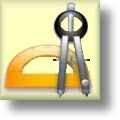Geometry Worksheets

## Triangle Worksheets

Here is a graphic preview for all of the Triangle Worksheets. You can select different variables to customize these Triangle Worksheets for your needs. The Triangle Worksheets are randomly created and will never repeat so you have an endless supply of quality Triangle Worksheets to use in the classroom or at home. We have a triangle fact sheet, identifying triangles, area and perimeters, the triangle inequality theorem, triangle inequalities of angles and angles, triangle angle sum, the exterior angle theorem, angle bisectors, median of triangles, finding a centroid from a graph and a set of vertices for your use. Our Triangle Worksheets are free to download, easy to use, and very flexible.

These Triangle Worksheets are a great resource for children in 5th, 6th Grade, 7th Grade, and 8th Grade.

Click here for a Detailed Description of all the Triangle Worksheets.

## Quick Link for All Triangle Worksheets

Click the image to be taken to that Triangles Worksheets.

##### Triangle FactsTriangle Worksheets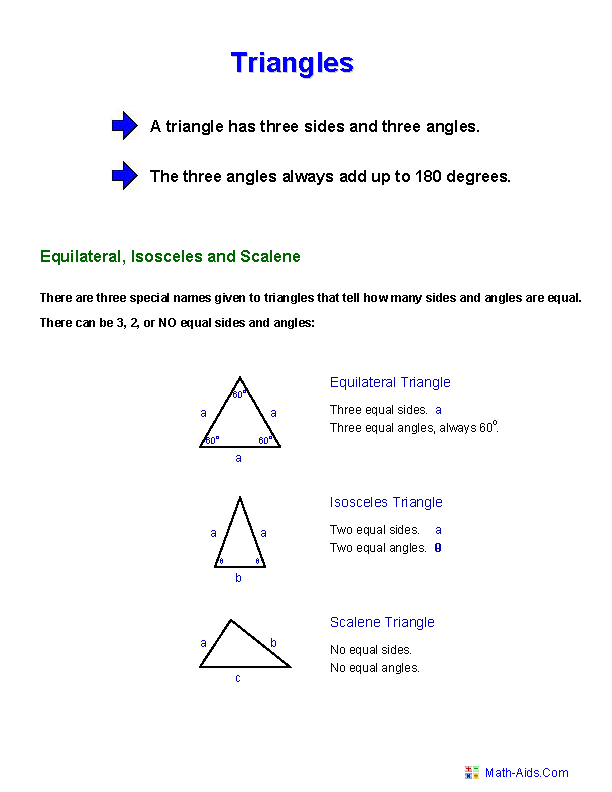##### Identify TrianglesTriangle Worksheets##### Area and Perimeter ofTriangles Worksheets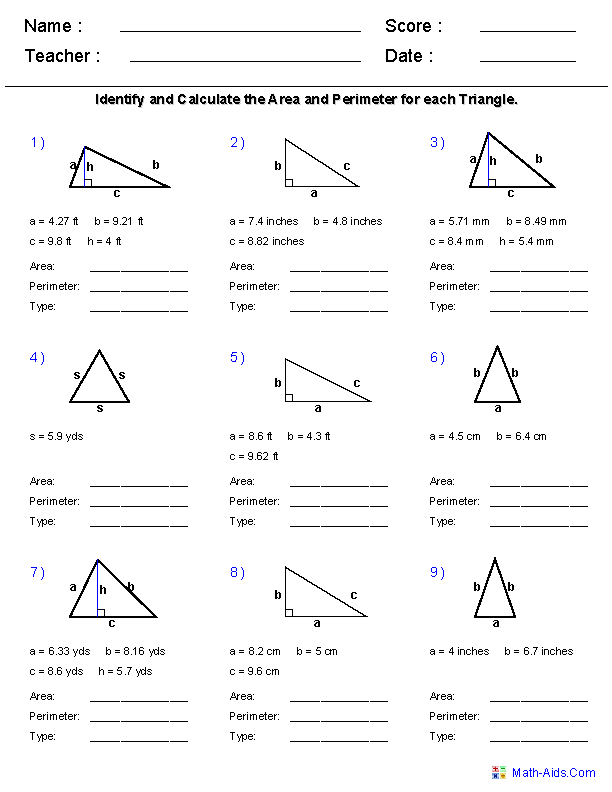##### The Triangle InequalityTheorem Worksheets##### Triangle Inequalities of AnglesTriangle Worksheets##### Triangle Inequalities of SidesTriangle Worksheets##### Triangle Angle SumTriangle Worksheets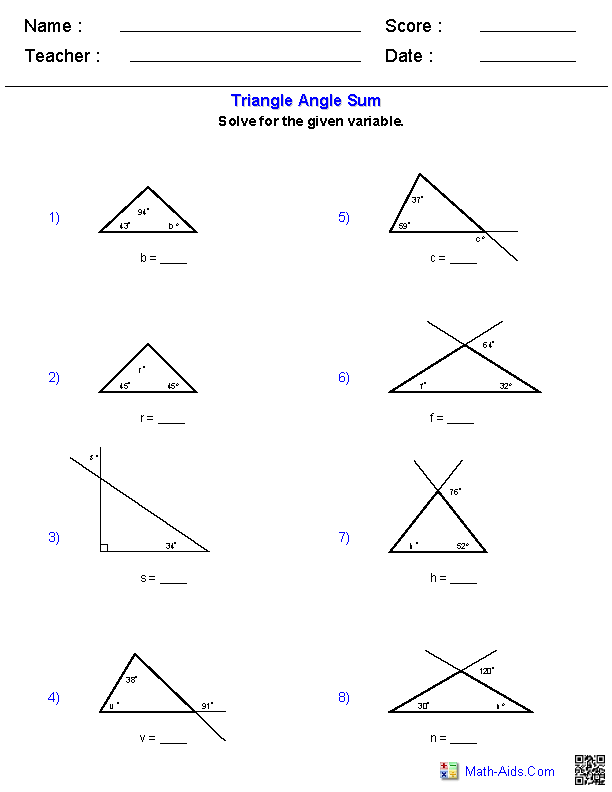##### The Exterior Angle TheoremTriangle Worksheets##### Angle Bisectors WorksheetsTriangle Worksheets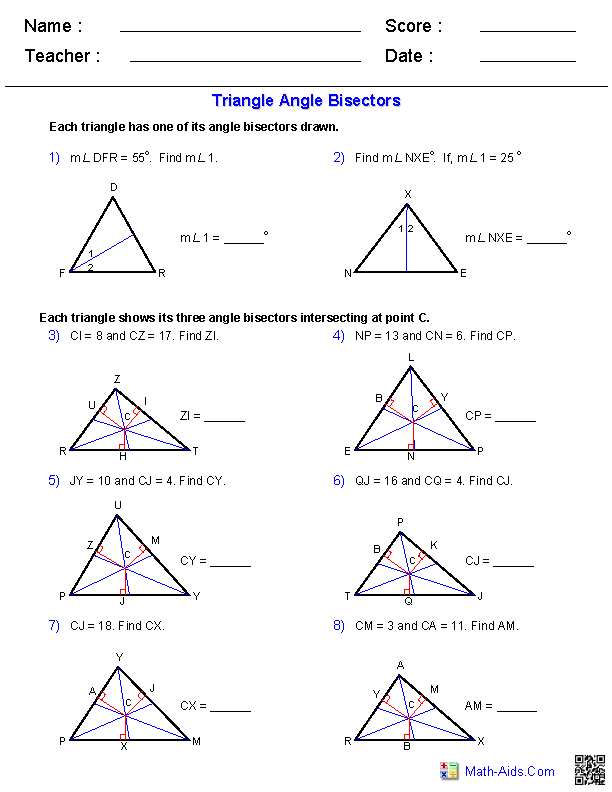##### Medians of TrianglesTriangle Worksheets##### Find the Centroidfrom a Graph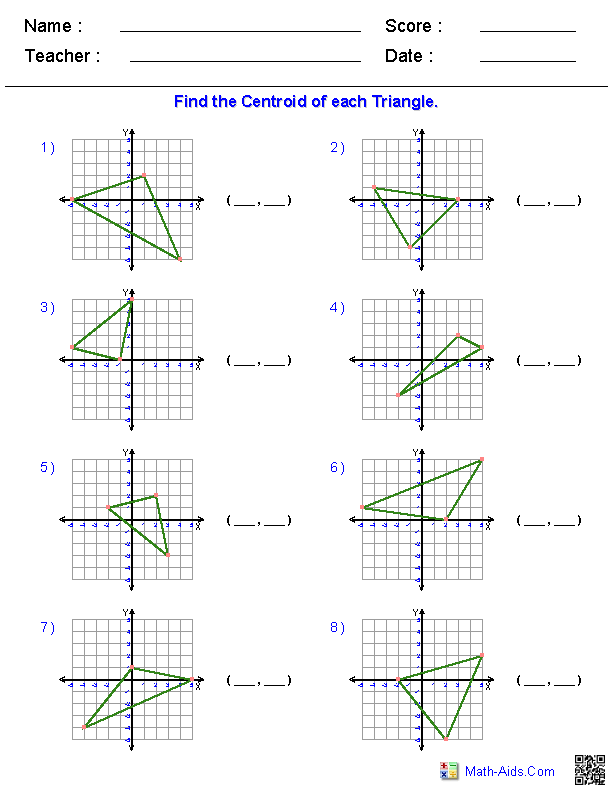##### Find the Centroidfrom Vertices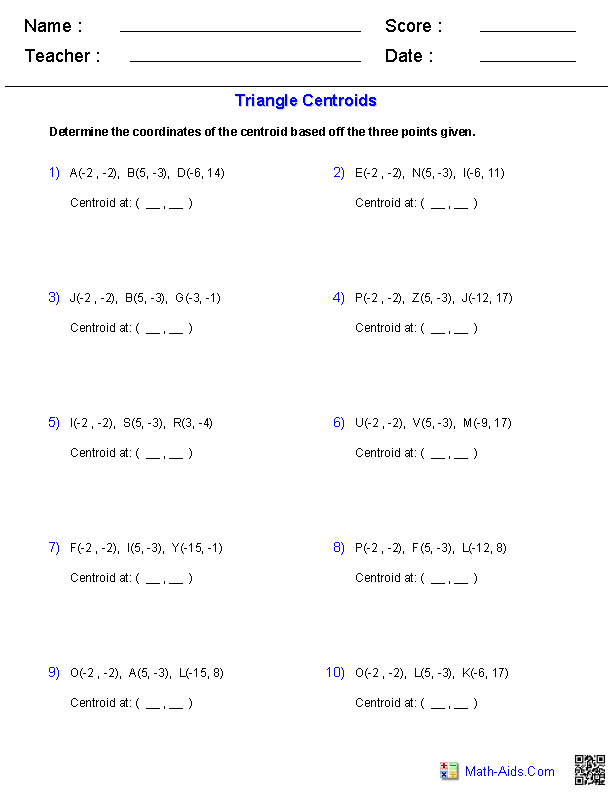Recommended Videos

## Detailed Description for All Triangle Worksheets

Triangle Facts Worksheets
This Triangle Worksheet will produce a useful definitions, facts and formulas handout for the students. This worksheet is a great resources for the 5th, 6th Grade, 7th Grade, and 8th Grade.

Identify Triangles Worksheets
This Triangle Worksheet will produce twelve problems for identifying different types of triangles. You may select equilateral, right scalene, right isosceles, obtuse scalene, obtuse isosceles, acute scalene and acute isosceles. This worksheet is a great resources for the 5th, 6th Grade, 7th Grade, and 8th Grade.

Area and Perimeter of Triangles Worksheets
This Triangle Worksheet will produce nine problems for solving the area and perimeter of different types of triangles. This worksheet is a great resources for the 5th, 6th Grade, 7th Grade, and 8th Grade.

Triangle Inequality Theorem Worksheets
This Triangle Worksheet will produce triangle inequality theorem problems. You can choose between between whole numbers or decimal numbers for this worksheet. This worksheet is a great resource for the 5th, 6th Grade, 7th Grade, and 8th Grade.

Triangle Inequalities of Angles Worksheets
This Triangle Worksheet will produce triangle angle inequality problems. This worksheet is a great resource for the 5th, 6th Grade, 7th Grade, and 8th Grade.

Triangle Inequalities of Sides Worksheets
This Triangle Worksheet will produce triangle side inequality problems. This worksheet is a great resource for the 5th, 6th Grade, 7th Grade, and 8th Grade.

Triangle Angle Sum Worksheets
This Triangle Worksheet will produce triangle angle sum problems. You can choose between interior and exterior angles, as well as an algebraic expression for the unknown angle. This worksheet is a great resource for the 5th, 6th Grade, 7th Grade, and 8th Grade.

The Exterior Angle Theorem Worksheet
This Triangle Worksheet will produce exterior angle theorem problems. You can choose a single variable or an algebraic expression for the unknown angle. This worksheet is a great resource for the 5th, 6th Grade, 7th Grade, and 8th Grade.

Triangle Angle Bisectors Worksheets
This Triangle Worksheet will produce angle bisector problems. This worksheet is a great resource for the 5th, 6th Grade, 7th Grade, and 8th Grade.

Medians of Triangles Worksheets
This Triangle Worksheet will produce median problems. You may select the problem types to be integers, decimals, or algebraic expression. This worksheet is a great resource for the 5th, 6th Grade, 7th Grade, and 8th Grade.

Find the Centroid from a Graph Worksheets
This Triangle Worksheet will produce problems to calculate the centroid from a graph. This worksheet is a great resource for the 5th, 6th Grade, 7th Grade, and 8th Grade.

Find the Centroid from Vertices Worksheets
This Triangle Worksheet will produce problems where you find the centroid from a the vertices of a triangle. This worksheet is a great resource for the 5th, 6th Grade, 7th Grade, and 8th Grade.

Click here for More Geometry WorksheetsMath-Aids.Com.  All rights reserved. Geometry Worksheets